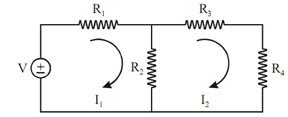## Nodal and Mesh Analysis Assignment Help

Assignment Help: >> Dc Circuits - Nodal and Mesh Analysis

Nodal and Mesh Analysis:

Kirchhoff's voltage law is utilized to find out the value of current in the multi loop circuit. For this purpose, Maxwell's loop method is utilized as explained by an example given below.

Figure shows the circuit having two loops and one voltage source in first loop.Figure: Maxwell's Loop Method

Suppose, two loop currents flowing in the directions illustrated in Figure. Now we may write the KVL equations for each loop.

KVL Equation for First Loop

∑ Voltage rise = ∑ Voltage drops

V = R1 I1  + R2  (I1  - I2 )

= ( R1  + R2 ) I1  - R2 I 2      ---------- (i)

KVL Equation in Second Loop

0 = R2  ( I2  - I1 ) + R3 I2  + R4 I2

=- R2 I1  + (R2  + R3  + R4 ) I2                  ------------(ii)

After solving out equations, we may find two loop currents I1 and I2. Voltage division rule is also one of the applications of KVL.